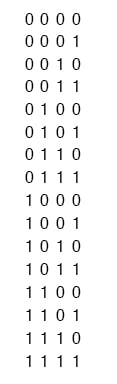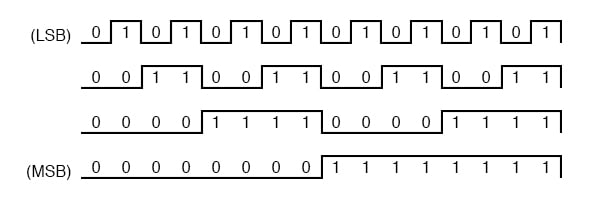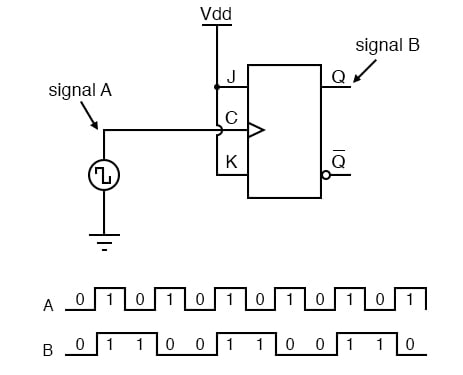Vol. Digital Circuits
Chapter 11 Sequential Circuits

# Binary Count Sequence

If we examine a four-bit binary count sequence from 0000 to 1111, a definite pattern will be evident in the “oscillations” of the bits between 0 and 1:Note how the least significant bit (LSB) toggles between 0 and 1 for every step in the count sequence, while each succeeding bit toggles at one-half the frequency of the one before it.

The most significant bit (MSB) only toggles once during the entire sixteen-step count sequence: at the transition between 7 (0111) and 8 (1000).

If we wanted to design a digital circuit to “count” in four-bit binary, all we would have to do is design a series of frequency divider circuits, each circuit dividing the frequency of a square-wave pulse by a factor of 2:J-K flip-flops are ideally suited for this task, because they have the ability to “toggle” their output state at the command of a clock pulse when both J and K inputs are made “high” (1):If we consider the two signals (A and B) in this circuit to represent two bits of a binary number, signal A being the LSB and signal B being the MSB, we see that the count sequence is backward: from 11 to 10 to 01 to 00 and back again to 11.

Although it might not be counting in the direction we might have assumed, at least it counts!

The following sections explore different types of counter circuits, all made with J-K flip-flops, and all based on the exploitation of that flip-flop’s toggle mode of operation.

REVIEW:

• Binary count sequences follow a pattern of octave frequency division: the frequency of oscillation for each bit, from LSB to MSB, follows a divide-by-two pattern. In other words, the LSB will oscillate at the highest frequency, followed by the next bit at one-half the LSB’s frequency, and the next bit at one-half the frequency of the bit before it, etc.
• Circuits may be built that “count” in a binary sequence, using J-K flip-flops set up in the “toggle” mode.

RELATED WORKSHEETS:

#### Lessons in Electric Circuits

Published under the terms and conditions of the Design Science License